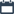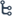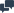## Friday, October 3010/30/2015No comments

## Outrage has Followed Student Being Marked Down for Claiming 5+5+5=15An online post of a student's maths quiz has caused a firestorm after it showed the student was marked down even though he gave the correct answers.

The quiz, posted to Reddit, caused outrage because the student was marked down by his teacher purely because he didn't get the answers using the means his teacher wanted. The first question had asked the student to calculate 5 x 3 using repeated addition. The student answered 5+5+5 = 15, but this was marked incorrect by the teacher who advised the accurate answer was 3+3+3+3+3=15.

Question two was equally as contentious with the student being asked to draw an array to solve 4x6.

For their answer, the student drew six rows of four dashes, but this was marked incorrect by the teacher who advised the correct answer was four rows of six dashes.New York high school math and physics teacher Frank Noschese said the questions were part of the Common Core standards — an educational initiative in the US detailing what students should know in English and maths at the end of each grade.

“The standards just lay out what kids should know and be able to do, not actual lessons. If the teacher specifically said ‘5x3 means five groups of three and 4x6 means four groups of six’ these answers are wrong because of the teacher’s forced interpretation. But mathematically, what the kid did is also valid. Kids likely know that five groups of three is equal to three groups of five.”
Do you think the answer was correct?